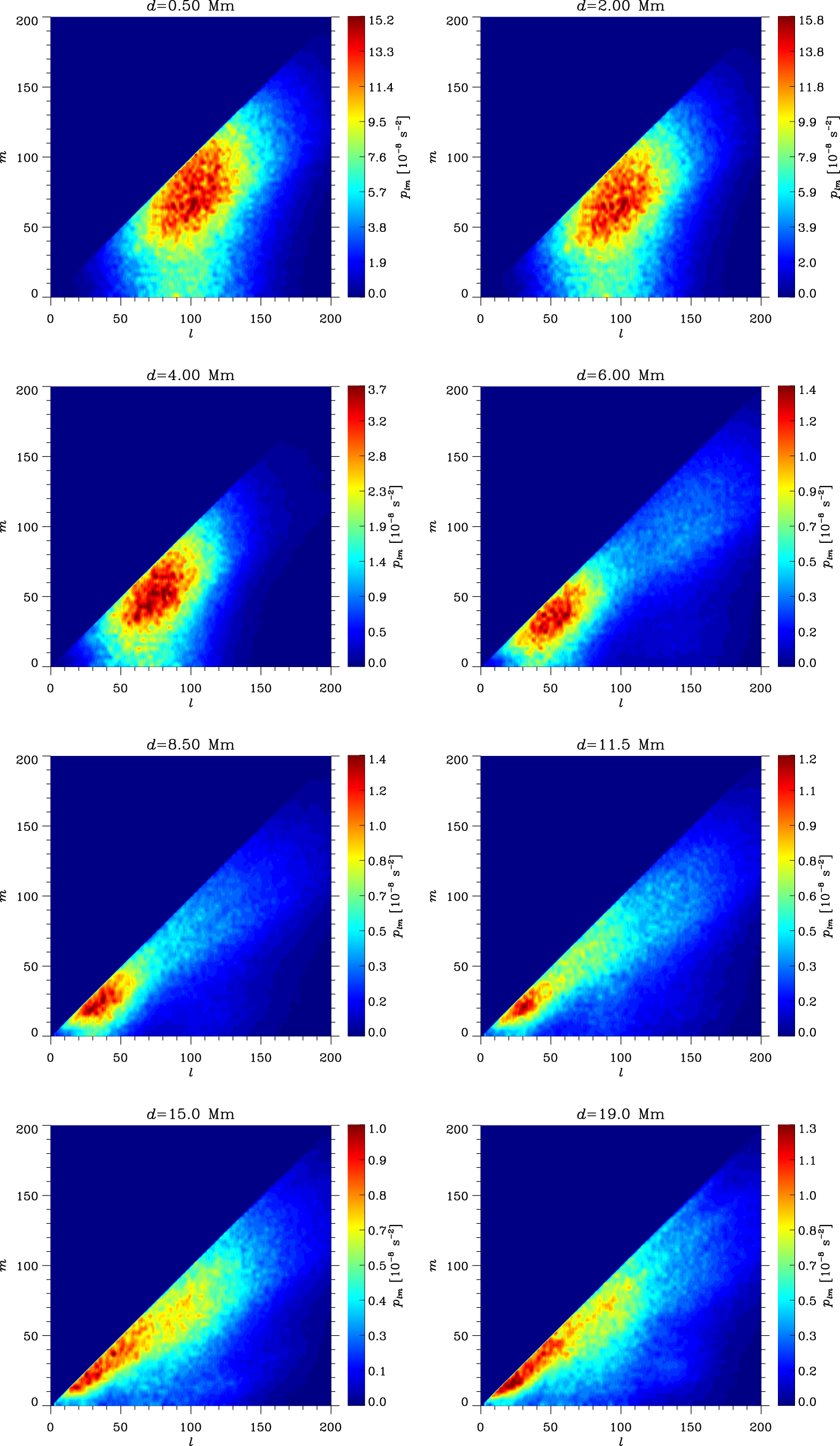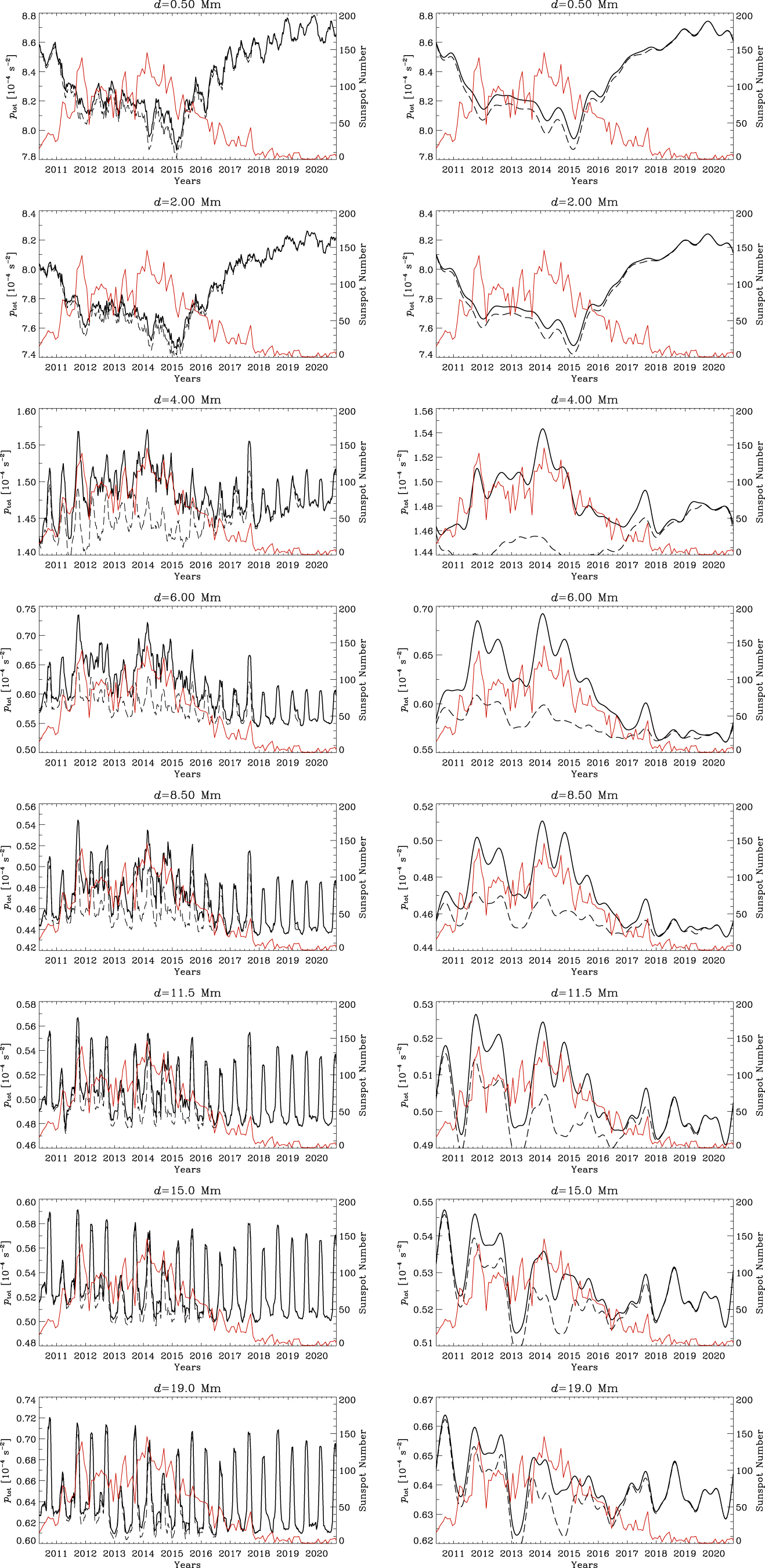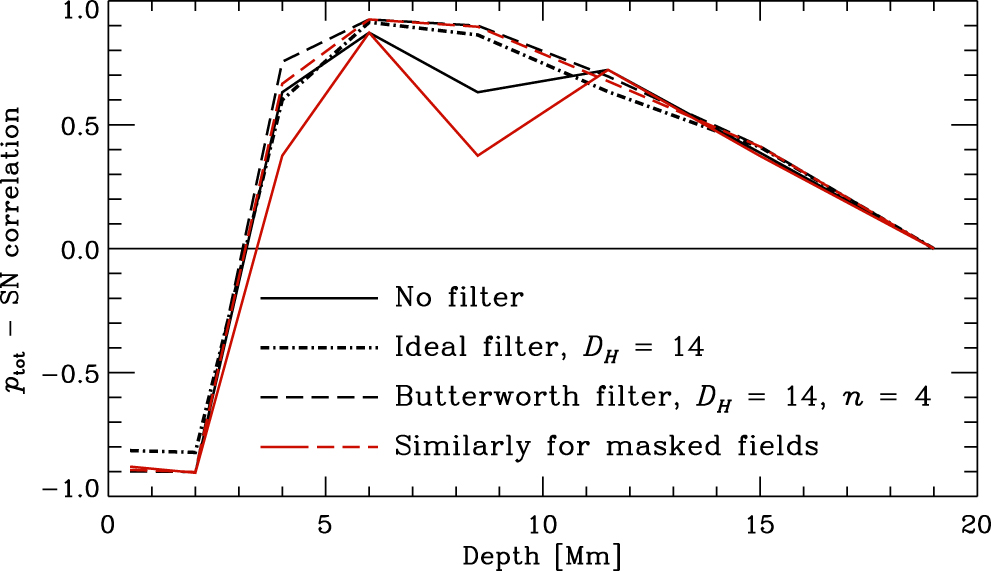# 189. Spatial Scales and Time Variation of Solar Subsurface Convection

Contributed by Alexander Getling. Posted on October 31, 2022

A. V. Getling1 & A. G. Kosovichev2
1 Skobeltsyn Institute of Nuclear Physics, Lomonosov Moscow State University, Moscow, Russia
2 New Jersey Institute of Technology, NJ 07102, USA

We study the spatiotemporal structure of subphotospheric convection using the full-disk horizontal-velocity maps obtained using the time–distance helioseismology, based on the data of Helioseismic and Magnetic Imager onboard the Solar Dynamics Observatory from May 2010 to September 2020. Specifically, we construct the spatial spectra of the horizontal velocity fields at the depths range of d=0–18 Mm and analyze the variations of the spectra with depth and time in the course of the solar activity cycle.

We use the data for the horizontal velocities on a grid of 1026 × 1026 pixels, with a spatial sampling interval of 0.12° in both longitude and latitude and an 8-hr time cadence. We calculate the field of the horizontal-velocity divergence and represent it as a polynomial expansion in spherical harmonics of angular degree l and azimuthal order m with llmax, where lmax is a properly chosen upper spectral boundary and assumed to be 200, and amplitudes Alm.Figure 1| Depth variations of a sample power spectra of the divergence of the velocity field smoothed with a 17.5 Mm window, plm, with lmax = 200, obtained by 45-day averaging over the low-activity period from 2019 December 21 to 2020 February 4. The depth values are indicated at the top of each panel.

We apply the running-averaging procedure with a 45-day window to the power spectra plm=|Alm|2. According to Parseval’s theorem, the integrated flow power is$p_{\mathrm{tot}} = \sum_{l=0}^{l_{\mathrm{max}}} \sum_{m=-l}^{m=l}|A_{lm}|^2$
The spatial scales of the field components represented by the harmonics of a given degree, l, can be estimated using Jeans’s formula$\lambda= 2\pi r_\odot / \sqrt{l(l+1)}$.

A typical series of power spectra for an individual 45-day averaging interval and different depths is shown in Figure 1. It can be seen that the main spectral peak shifts to long wavelengths with depth. The most energetic harmonics in the upper layers have l ~ 100–120 (λ ~ 30 Mm, supergranular scales); at large depths, l ~15–30 (λ values of up to ~ 300 Mm, giant-cell scales). We have to note the following feature: as d increases, the spectral peak approaches the line l = m, which corresponds to sectorial (θ-independent) harmonics. Therefore, the large-scale cells exhibit a tendency to a banana shape.Figure 2| Time variations of the total power of all the harmonics of the unmasked fields (solid curves) and masked fields (dashed curves) for the depths indicated at the top of each panel. Left: unfiltered; right: filtered by applying the Butterworth filter, with fH= 14, n = 4. The red curve in each panel represents the monthly average sunspot number.

The integrated flow power, ptot, undergoes variation in the activity cycle, which becomes progressively noisier with depth because of the presence of the half-year harmonic due to changes in the inclination of the Sun’s rotational axis to the line of sight (Fig. 2, left column). To isolate the physically meaningful component of the variation, we employ a low-pass spectral-filtering procedure, either cutting the spectrum of the variation at a frequency of νH=0.05 μHz or multiplying it by the Butterworth filter$H(\nu)=1/[1+(\nu/\nu_H )^{2n}]$, n = 4, and performing an inverse Fourier transform. The filtered variation (Fig. 2, right column) is anticorrelated with the sunspot number in the upper layers and positively correlated in the deeper layers. The depth variation of the correlation coefficient is shown in Fig. 3.

To assess the possible effect of magnetic fields on the calculated variation of ptot, this variation was also calculated for “masked” fields in which the divergence values in the areas of strong magnetic fields were replaced with its mean value. The results are shown with dashed curves in Fig. 2 and red curves in Fig. 3.Figure 3| The coefficient of correlation between the integrated flow power and the sunspot number as a function of depth.

Thus, we see that the subsurface flow represents a superposition of multiscale convective structures traced at various levels in the upper convection zone. The range of flow scales is fairly wide in shallow layers. As the depth increases, this range narrows, and the main peak shifts approaching giant-cell scale. A tendency toward the formation of banana-shaped cells is observed. The integrated power is anticorrelated with the solar-activity level in near-surface layers and positively correlated in deeper layer. The correlation reaches its maximum at depths of ~ 6 Mm.

For more detail, see Ref.  at https://ui.adsabs.harvard.edu/abs/2022ApJ…937…41G.

### References

 Scherrer, P. H., Schou, J., Bush, R. I., et al. 2012, Solar Phys., 275, 207
 Zhao, J., Couvidat, S., Bogart, R. S., et al. 2012, Solar Phys., 275, 375
 Getling, A.V., and Kosovichev, A.G. 2022, Astrophys. J., 937, 41